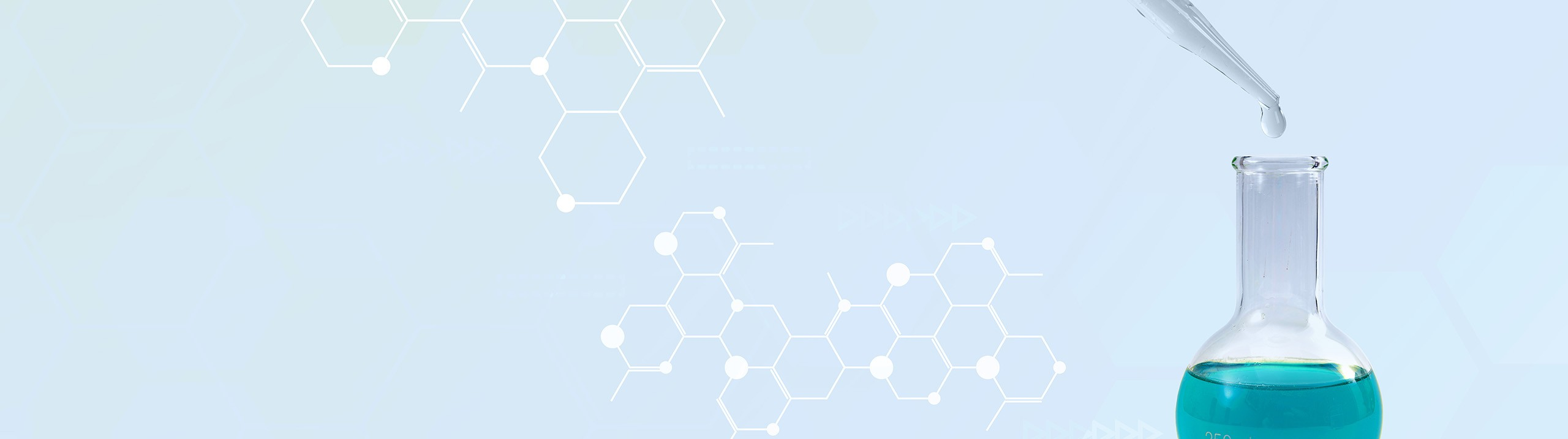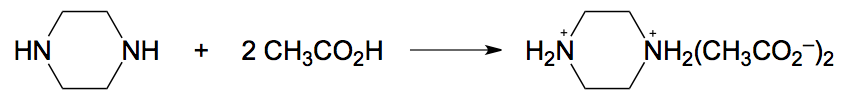## Analytical Chemistry

Learn the toughest concepts covered in your Analytical Chemistry class with step-by-step video tutorials and practice problems.

16. Electroanalytical Techniques

# Gravimetric Analysis

In Gravimetric Analysis the mass of a product in a chemical reaction is used to calculate the amount of the original analyte.

Introduction to Gravimetric Analysis
1
example

## Electrogravimetric Analysis Example 13m
Play a video:
2
example

## Electrogravimetric Analysis Practice4m
Play a video:
So here in this practice question, it states the iron and a 1.1530 g sample of iron ore is precipitated as iron three oxide connected to an unknown amount of water molecules by the addition of ammonia. The residue is ignited. High temperatures to give 30.6310 g of pure iron three oxide, calculate the weight percent of iron in the ore. Alright, So they want us to determine the mass percent or weight percent of iron. Within our sample of iron ore. We're gonna say here that the weight percent or mass percent of iron equals the grams of iron divided by the mass of the ore. So grams of ore. Now we already know how much of our iron ore we have. We're told that in the very first sentence. So we're gonna plug that in. So that's 1.1530 g of your. What we need to do next though is we need to determine the amount of our iron an elite. And the way we're able to determine that is by using the mass of the product that we obtained from the chemical reaction. Alright, so we're gonna take these .6310 g of iron to Iron three oxide. We're gonna change it into moles. So we're gonna say one mole of iron, three oxide. How many grams are involved While it's composed of two irons and three oxygen's. So the combined molar mass of it would come out to 159.687 g Here, the grams of iron three oxide cancel out And we're left with moles of iron three oxide. Now, we need to make the jump from uh moles of Iron three oxide to just moles of iron. If we look at our balanced equation, we can see that it's a 1-1 relationship. We can assume that actually a 1-2. So I wanted to relationship between Iron three Oxide and Iron three. And we can say that that iron three originates from the pure non charged iron form. So we can make that that jump. So we're gonna say here that for every one mole of Iron three oxide, We have two moles of iron three ion. And by extension, two moles of iron. So these moles cancel out. And then we're gonna say here that we need grams of iron. So we're gonna stay here for every one mole of iron. Its atomic mass according to periodic table is 55.845g. Most of iron cancel out and at the end will have .44134 g of iron. Take those grams of iron and plug them in. Multiply this by 100 to get our percent. And when we do that, we get approximately 38.3% as the weight percent of our iron within this or sample. So again, with the information from the amount of product obtain were able to gain information on the amount of our original an allied and by extension in this case the weight percent of the an elite. So now that we've seen these two problems, let's move on to our next question dealing with gravimetric analysis.
Electrogravimetric Analysis Calculations
3
example

## Electrogravimetric Analysis Calculations Example 14m
Play a video:
So here in this example, it states the reaction between piper zine and acetic acid creates an adult product known as piper scene di acetate. So here we have piper's in one mole piper seen reacting with two moles of acetic acid that produces this larger product here, one mole of it. Now here it says a 7.50 g sample of impure pipe ursine contained 83.1% piper zine. How many grams of piper scene di acetate would be formed in the process. Alright, so here they're actually asking us to determine the amount of our product, the grams of this. And they're giving us basically the grams of the impure pipe racine and its mass percent. With this information, we should be able to determine the mass of the product. So up to this point, we've been given the mass of our product and been able to use that to find the original. An elite in this case. Now we're given the an elite and we're just trying to figure out how much product we have. So you can approach this in the same way as any dimensional analysis question coupled with soy geometry. So we have 7.50 g of our impure pipe ursine. So that actually represents grams of our solution. Because if it was pure piper seen, then that would be 7.50 g of it. Now they're giving us mass percent of piper seen. What this is really saying is that for every 83.1 g of piper's scene, There are 100 g of our solution. And by lining it up and setting it up. This way we can see that the grams of solution will cancel out now that we have our grams of piper's scene. We can just use geometry to go from grams of piper's in two moles of piper's seen two moles of our product. Alright, so pipe racine itself. Remember that every corner is a carbon. Carbon itself Must make four bonds and those carbons to make four bonds, they have hydrogen that are not visible. Okay, so this formula here has four carbons. It has 10 hydrogen and two nitrogen. When we add all of that up, that will give us the molar mass of piper scene. So when we add all that up, that gives us 86.136 g. And then that's for every one mole of it grams of pipers and cancel out. And now we're gonna figure out the moles of our pipers in di acetate. We can see from the balanced equation up above that, it's a 1 to 1 relationship. So for every one mole of piper's scene, we have one mole of our product and then one mole. And to make things easier, I'll just abbreviated as P. A. Or P. D. Actually for pipers in di acetate moles cancel out. I now have moles of my product. So all we have to do now is just convert those moles into grams. When we add up all the portions of the structure, its combined mass comes out to 206.236 g For every one mole moles cancel out. So at the end we're gonna have grams of piper's in di acetate. So when we work that out, we're gonna get 14.9 g. And here my answer has three sig figs because 7.50 has three sig figs. And this percentage here has four sig figs. Right? We always go with the least number of sig figs. So 14 point now will be our best answer. Now, just remember gravimetric analysis were usually given the mass of our product and with that we're able to determine the amount of our original. An elite. In this case, I'm giving us information on the an elite and use that to figure out the amount of product now that we've seen this question. Move on to the next4
example

## Electrogravimetric Analysis Calculations Example 26m
Play a video: